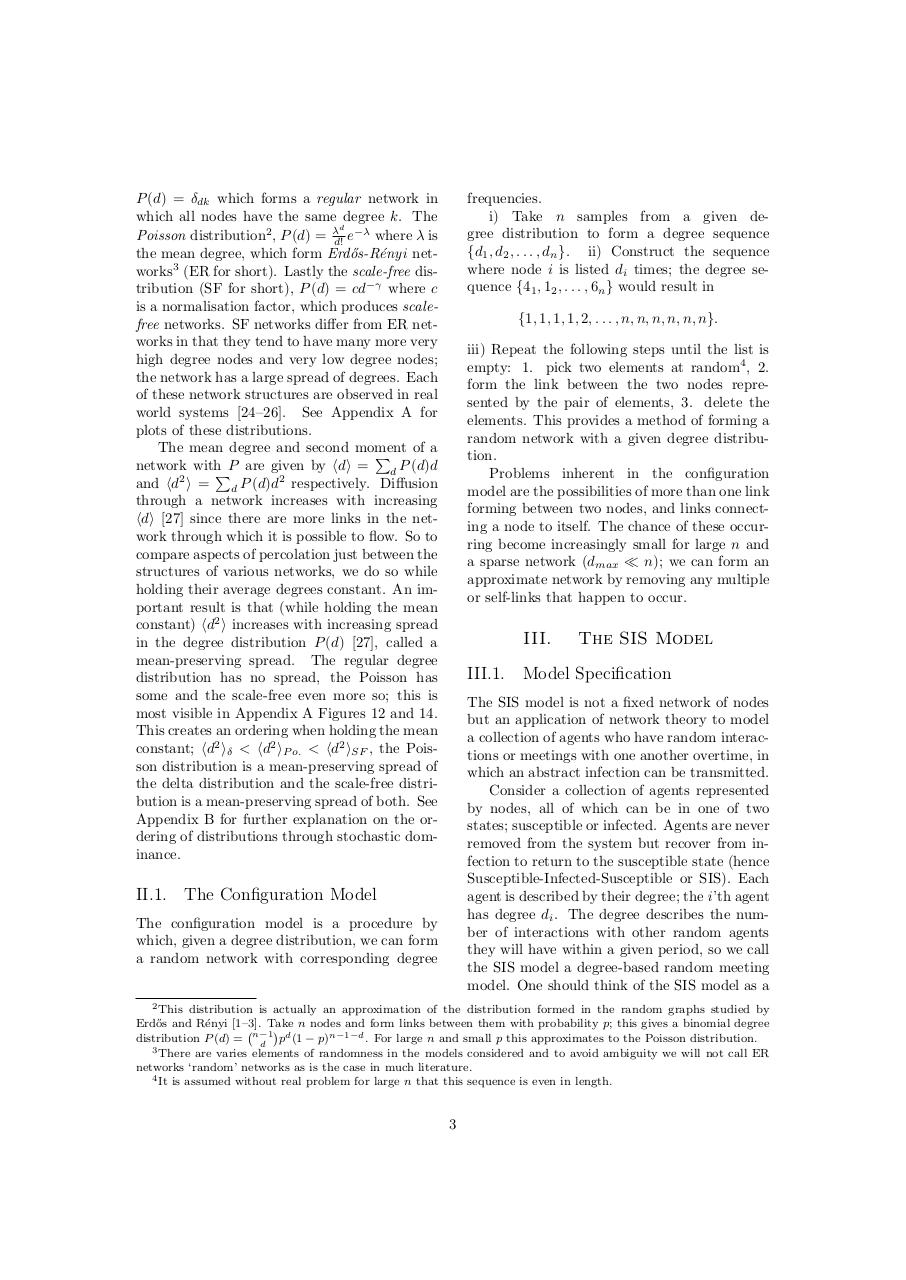# PDF Archive

Easily share your PDF documents with your contacts, on the Web and Social Networks.

## Adaptive Percolation Daniel Burkhardt Cerigo.pdfPage 1 2 34524

#### Text preview

P (d) = dk which forms a regular network in
which all nodes have the same degree k. The
d
Poisson distribution2 , P (d) = d! e where is
the mean degree, which form Erd˝
os-R´enyi networks3 (ER for short). Lastly the scale-free distribution (SF for short), P (d) = cd
where c
is a normalisation factor, which produces scalefree networks. SF networks di↵er from ER networks in that they tend to have many more very
high degree nodes and very low degree nodes;
the network has a large spread of degrees. Each
of these network structures are observed in real
world systems [24–26]. See Appendix A for
plots of these distributions.
The mean degree and second moment
of a
P
network withPP are given by hdi = d P (d)d
and hd2 i = d P (d)d2 respectively. Di↵usion
through a network increases with increasing
hdi  since there are more links in the network through which it is possible to flow. So to
compare aspects of percolation just between the
structures of various networks, we do so while
holding their average degrees constant. An important result is that (while holding the mean
constant) hd2 i increases with increasing spread
in the degree distribution P (d) , called a
distribution has no spread, the Poisson has
some and the scale-free even more so; this is
most visible in Appendix A Figures 12 and 14.
This creates an ordering when holding the mean
constant; hd2 i &lt; hd2 iP o. &lt; hd2 iSF , the Poisson distribution is a mean-preserving spread of
the delta distribution and the scale-free distribution is a mean-preserving spread of both. See
Appendix B for further explanation on the ordering of distributions through stochastic dominance.

frequencies.
i) Take n samples from a given degree distribution to form a degree sequence
{d1 , d2 , . . . , dn }. ii) Construct the sequence
where node i is listed di times; the degree sequence {41 , 12 , . . . , 6n } would result in
{1, 1, 1, 1, 2, . . . , n, n, n, n, n, n}.
iii) Repeat the following steps until the list is
empty: 1. pick two elements at random4 , 2.
form the link between the two nodes represented by the pair of elements, 3. delete the
elements. This provides a method of forming a
random network with a given degree distribution.
Problems inherent in the configuration
model are the possibilities of more than one link
forming between two nodes, and links connecting a node to itself. The chance of these occurring become increasingly small for large n and
a sparse network (dmax ⌧ n); we can form an
approximate network by removing any multiple
or self-links that happen to occur.

III.

The SIS Model

III.1. Model Specification
The SIS model is not a fixed network of nodes
but an application of network theory to model
a collection of agents who have random interactions or meetings with one another overtime, in
which an abstract infection can be transmitted.
Consider a collection of agents represented
by nodes, all of which can be in one of two
states; susceptible or infected. Agents are never
removed from the system but recover from infection to return to the susceptible state (hence
Susceptible-Infected-Susceptible or SIS). Each
agent is described by their degree; the i ’th agent
has degree di . The degree describes the number of interactions with other random agents
they will have within a given period, so we call
the SIS model a degree-based random meeting
model. One should think of the SIS model as a

II.1. The Configuration Model
The configuration model is a procedure by
which, given a degree distribution, we can form
a random network with corresponding degree

2 This distribution is actually an approximation of the distribution formed in the random graphs studied by
Erd˝
os and R´
enyi [1–3]. Take n nodes and form links between them with probability p; this gives a binomial degree
distribution P (d) = n d 1 pd (1 p)n 1 d . For large n and small p this approximates to the Poisson distribution.
3 There are varies elements of randomness in the models considered and to avoid ambiguity we will not call ER
networks ‘random’ networks as is the case in much literature.
4 It is assumed without real problem for large n that this sequence is even in length.

3